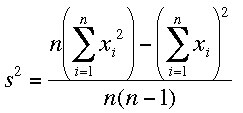SEARCH HOMEMath Central Quandaries & QueriesHi I searched the listings but couldn't find what i am looking for. I have a data set and i am allowed only 1 pass through it. At the end of this pass i must have the mean and standard deviation. Calculating the mean is easy as also demonstrated in your listings. I think the same can be done for standard deviation as well. But the doubt that i have is this :- Will the calculated standard deviation be sequence dependent ? i.e. if i change the order in which the data items enter the system, will the std deviation also change ?? Thanks in advance MurtazaMurtaza,

There are one pass expressions for the variance s2 and then the standard deviation is the square root of the variance. One such expression isIf you can keep a running total of the data values xi, the squares of the data values xi2 and the number of data values then you can assemble this expression after all the data is read once. This expression only uses the data values and the number of data values and not the order in which the values are read.

I hope this helps,
PennyMath Central is supported by the University of Regina and The Pacific Institute for the Mathematical Sciences.